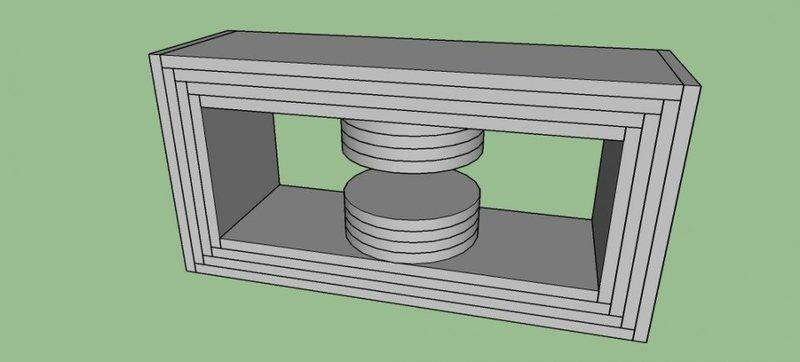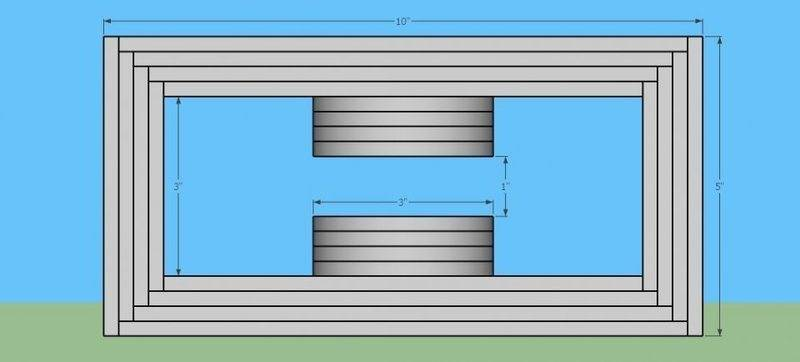# Calculating field strength of an electromagnet

I would like to build an electromagnet to accelerate electrons into a circle (a precursor to a mass spectrometer). I would like to calculate the field strength, but I don't know how because I would like to wind it out of aluminum strap salvaged from a welder, and I can only find formulas for solenoids (and I don't fully understand how the formulas are derived, and therefore can't find the formula myself). The core resembles an E core, but with a large air gap in the center leg where the electrons (and hopefully ions eventually) will pass through. The middle leg is also round, while the others are rectangular.

I can supply plans of the core if need be.

Hesch
Gold Member
I can supply plans of the core if need be.
Yes, that would be comfortable.

Generally Ampere's law is used to calculate the H-field ( also as for solenoids ):

circulation H⋅ds = N * I , where s is a circulation path.

This is the core. I would like to wind the magnet such that the maximum power I would reasonably put through it would be very close to the saturation of the core.It is made of mild steel, and is 3" deep.
The coils go around the circular parts and will be wound with aluminum strap.
The mean path should be 16" (0.41m)
The surface are the magnet is on is 3" diameter, with a 7.07 in^2 cross section.
Each outer leg has a 3 inch cross section.
Given a saturation flux for mild steel of 1.2 Teslas, I calculate the gap could be at a maximum of 1.02 Teslas.
Since the permeability of mild steel is 1.26e-4 H/m, it should have an AL value of 5.12e-5 H/n^2
I have to measure the thickness of the aluminum, and the insulation, to determine the winding density.

Hesch
Gold Member
The cross section areas are not the same along a circulating path, so you must divide this path into pieces represented by various magnetic "resistors".

In this way you can create an electric analogy to the magnetic circuit, for example by inserting a voltage source in the airgap, representing the desired H-field

Well, the Lorentz force due to the magnetic field must be equal to the centripetal force needed to keep the electrons in a circular path. This condition is qvB = mv^2/r, where the left hand side is the lofentz force and the right side is the centripetal force. q is the electron charge, v is the velocity of the electrons (determined by your accelerating potential) B is the magnetic field strength, m is the electron mass, and r is the radius of curvature you are looking for. so B = mv^2/(qvr).

Hopefully that gets you started. The best way to generate a magnetic field is to use a Helmholtz coil configuration, which produces are fairly uniform B-field.

Hesch
Gold Member
Given a saturation flux for mild steel of 1.2 Teslas, I calculate the gap could be at a maximum of 1.02 Teslas.
The best way to generate a magnetic field is to use a Helmholtz coil configuration
I think it's very hard to generate about 1 Tesla by a Helmholtz configuration with no core.

I think it's very hard to generate about 1 Tesla by a Helmholtz configuration with no core.
Actually, it doesn't take a large magnetic field to accelerate electrons into a circular path unless the electron energy is very large.

Thank you all who have replied. I have some numbers to crunch now. I think I'll ditch the aluminum strap, knowing that winding density is important, and instead use 18awg magnet wire. (I have maybe enough for one coil).

I was thinking, since the only way I currently know of to measure beta electron energy is to use a scintillation detector, could you conceivably build a beta spectrometer with this and measure the energy distribution from a reasonably radioactive source?